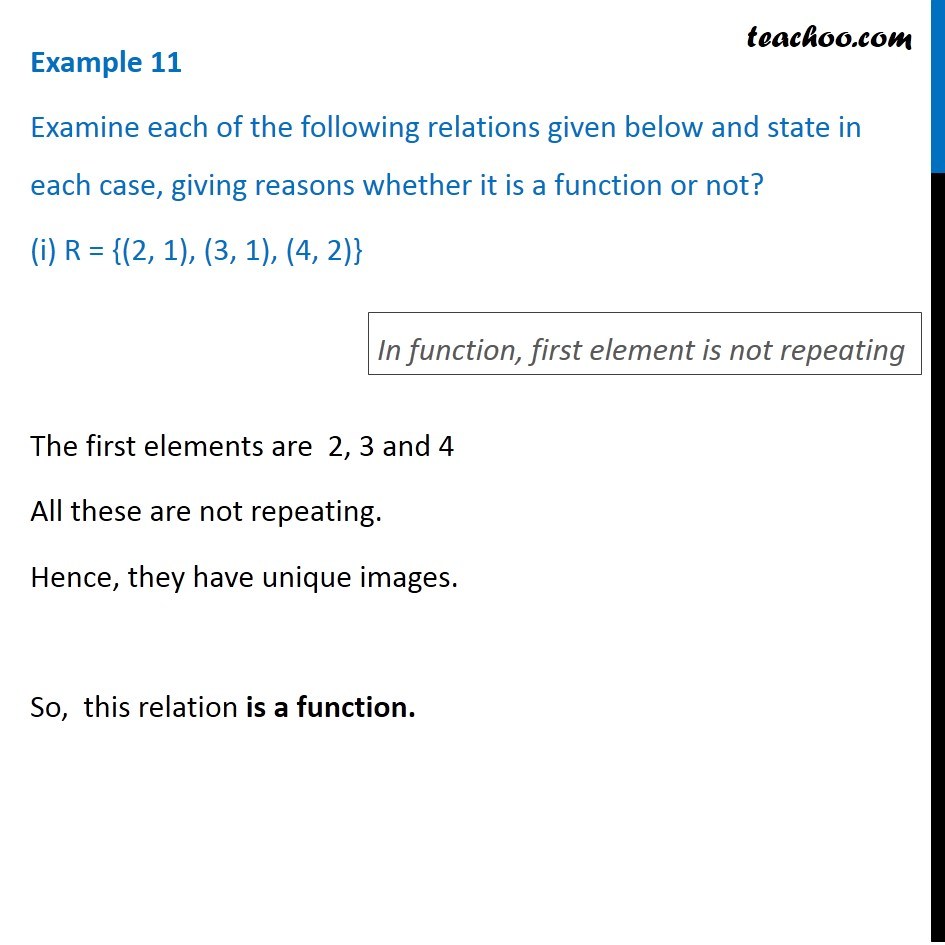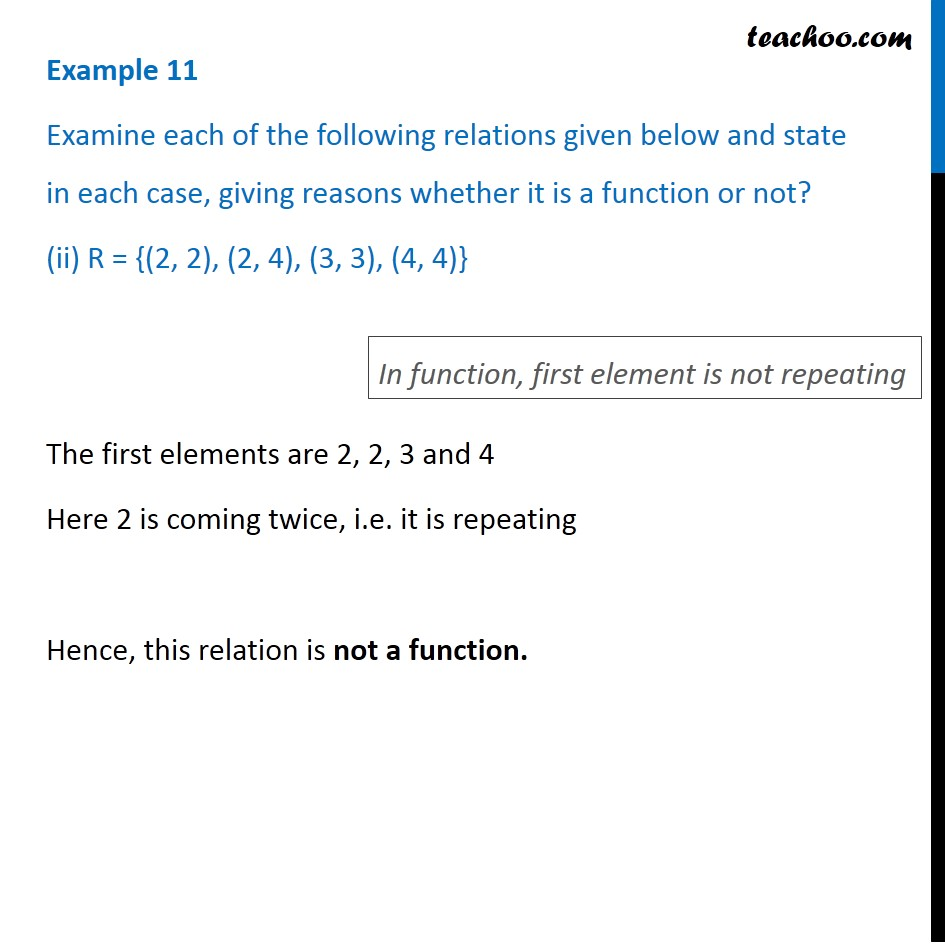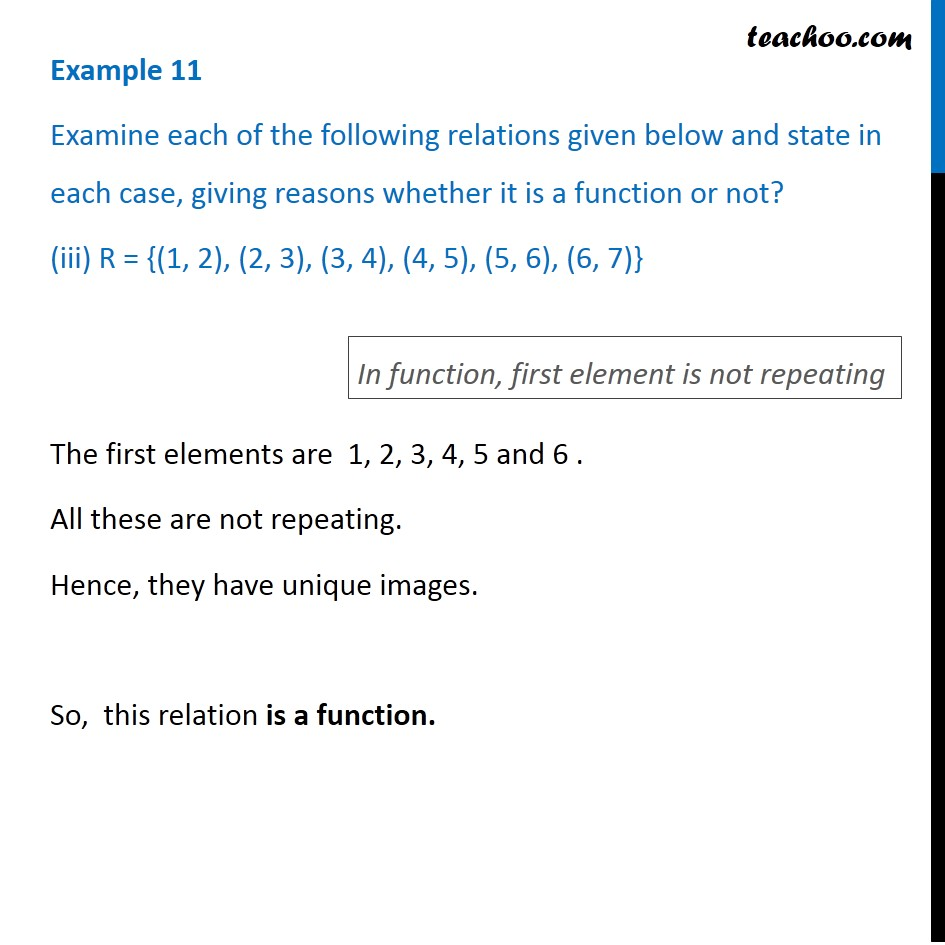Learn All Concepts of Chapter 2 Class 11 Relations and Function - FREE. Check - Relation and Function Class 11 - All Concepts1. Chapter 2 Class 11 Relations and Functions
2. Serial order wise
3. Examples

Transcript

Example 11 Examine each of the following relations given below and state in each case, giving reasons whether it is a function or not? (i) R = {(2, 1), (3, 1), (4, 2)} The first elements are 2, 3 and 4 All these are not repeating. Hence, they have unique images. So, this relation is a function. In function, first element is not repeating Example 11 Examine each of the following relations given below and state in each case, giving reasons whether it is a function or not? (ii) R = {(2, 2), (2, 4), (3, 3), (4, 4)} The first elements are 2, 2, 3 and 4 Here 2 is coming twice, i.e. it is repeating Hence, this relation is not a function. In function, first element is not repeating Example 11 Examine each of the following relations given below and state in each case, giving reasons whether it is a function or not? (iii) R = {(1, 2), (2, 3), (3, 4), (4, 5), (5, 6), (6, 7)} The first elements are 1, 2, 3, 4, 5 and 6 . All these are not repeating. Hence, they have unique images. So, this relation is a function. In function, first element is not repeating

Examples